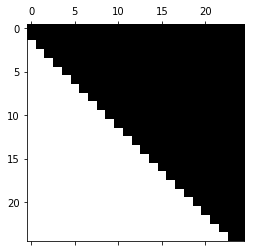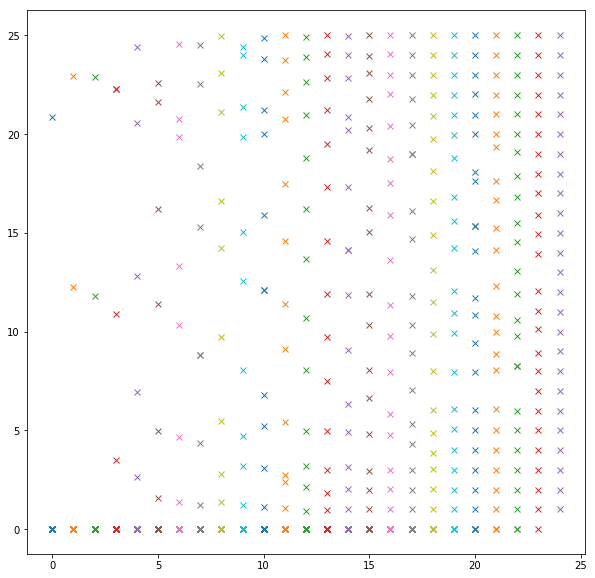# Arnoldi Iteration¶

In :
import numpy as np
import numpy.linalg as la

import matplotlib.pyplot as pt


Let us make a matrix with a defined set of eigenvalues and eigenvectors, given by eigvals and eigvecs.

In :
np.random.seed(40)

# Generate matrix with eigenvalues 1...25
n = 25
eigvals = np.linspace(1., n, n)
eigvecs = np.random.randn(n, n)
print(eigvals)

A = la.solve(eigvecs, np.dot(np.diag(eigvals), eigvecs))
print(la.eig(A))

[ 1.  2.  3.  4.  5.  6.  7.  8.  9. 10. 11. 12. 13. 14. 15. 16. 17. 18.
19. 20. 21. 22. 23. 24. 25.]
[25. 24. 23.  1.  2.  3. 22.  4. 21. 20.  5.  6.  7. 19. 18.  8.  9. 17.
16. 10. 11. 12. 15. 14. 13.]


## Initialization¶

Set up $Q$ and $H$:

In :
Q = np.zeros((n, n))
H = np.zeros((n, n))

k = 0


Pick a starting vector, normalize it

In :
x0 = np.random.randn(n)
x0 = x0/la.norm(x0)

# Poke it into the first column of Q
Q[:, k] = x0

del x0


Make a list to save arrays of Ritz values:

In :
ritz_values = []


## Algorithm¶

Carry out one iteration of Arnoldi iteration.

Run this cell in-place (Ctrl-Enter) until H is filled.

In :
print(k)

u = A @ Q[:, k]

# Carry out Gram-Schmidt on u against Q
for j in range(k+1):
qj = Q[:, j]
H[j,k] = qj @ u
u = u - H[j,k]*qj

if k+1 < n:
H[k+1, k] = la.norm(u)
Q[:, k+1] = u/H[k+1, k]

k += 1

pt.spy(H)

ritz_values.append(la.eig(H))

24Check that $Q^T A Q =H$:

In :
la.norm(Q.T @ A @ Q - H)/ la.norm(A)

Out:
5.694093461629727e-08

Check that Q is orthogonal:

In :
la.norm(Q.T @ Q - np.eye(n))

Out:
9.785682262092261e-08

## Plot convergence of Ritz values¶

Enable the Ritz value collection above to make this work.

In :
pt.figure(figsize=(10,10))
for i, rv in enumerate(ritz_values):
pt.plot([i] * len(rv), rv, "x")

/usr/local/lib/python3.7/site-packages/numpy/core/numeric.py:501: ComplexWarning: Casting complex values to real discards the imaginary part
return array(a, dtype, copy=False, order=order)In :
A = np.random.rand(2,2) + 1j*np.random.rand(2,2)
print(A)

[[0.23814271+0.34399174j 0.69827952+0.84920957j]
[0.82652298+0.93565511j 0.72234023+0.35809612j]]

In :
A.conj()

Out:
array([[0.23814271-0.34399174j, 0.69827952-0.84920957j],
[0.82652298-0.93565511j, 0.72234023-0.35809612j]])
In :
A.conj().T

Out:
array([[0.23814271-0.34399174j, 0.82652298-0.93565511j],
[0.69827952-0.84920957j, 0.72234023-0.35809612j]])
In :
import scipy.linalg as sla

In :
sla.